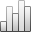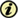Acta Stereologica

0351-580XMore Statisticssince 05 December 2013 :
View(s): 282 (1 ULiège)

### A further method to measure fractal dimension and the maximum property

(Volume 15 (1996) — Number 1 - Mathematical foundations of stereology - Feb. 1996)
Article#### Abstract

The fractal dimension of sets is used in praxis e.g. as a roughness parameter for surfaces, as a texture parameter or for classifying the complexity of line structures. An essential property of fractal dimension is that the most complex-structured part of a set determines tl1e dimension, mathematically described by the maximum property. The most popular methods to measure fractal dimension are the divider stepping method, the sausage method and the box-counting method. Measurements with these methods depend on the range of magnification and on the way the (necessary) linear regression is done. All these methods do not fulfill the maximum property. A new method based on the box counting theorem is represented here, called extended counting method. It avoids the linear regression, is increasing and even has a quasi maximum property, i.e. the maximum property is fulfilled except of a correction term, which tends to zero if the range of magnification tends to infinity. Several examples are shown demonstrating the method and its properties.

Keywords : complexity, box counting method, fractal dimension, maximum property, self-similar